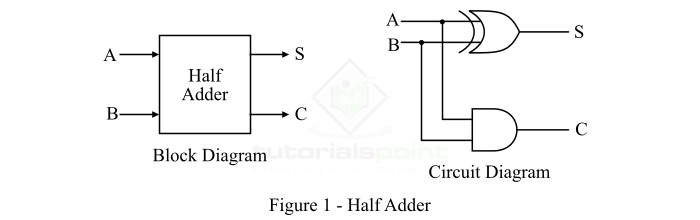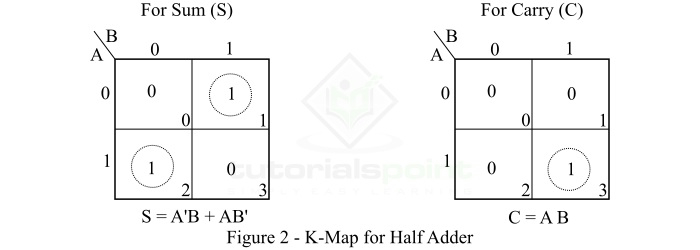# Half Adder in Digital Electronics

Addition is one of the most basic operations performed by different electronic devices like computers, calculators, etc. The electronic circuit that performs the addition of two or more numbers, more specifically binary numbers, is called as adder. Since, the logic circuits use binary number system to perform the operations, hence the adder is referred to as binary adder

Depending on the number of bits that the circuit can add, adders (or binary adders) are of two types −

In this article, we will discuss the half adder, its definition, circuit diagram, truth table, kmap, characteristic equations, and applications.

A combinational logic circuit which is designed to add two binary digits is called as a half adder. The half adder provides the output along with a carry value (if any). The half adder circuit is designed by connecting an EX-OR gate and one AND gate. It has two input terminals and two output terminals for sum and carry. The block diagram and circuit diagram of a half adder are shown in Figure-1.From the logic circuit diagram of half adder, it is clear that A and B are the two input bits, S is the output sum, and C is the output carry bit.

In the case of a half adder, the output of the EX-OR gate is the sum of two bits and the output of the AND gate is the carry. Although, the carry obtained in one addition will not be forwarded in the next addition because of this it is known as half adder.

Half adder adds two binary digits according to the rules of binary addition. These rules are as follows −

      0 + 0 = 0
0 + 1 = 1
1 + 0 = 1
1 + 1 = 10 (Sum = 0 & Carry = 1)


According to these rules of binary addition, we can see that the first three operations produce a sum whose length is one digit, whereas in the case of last operation (1 and 1), the sum consists of two digits. Here, the MSB (most significant bit) of this result is called a carry (which is 1) and the LSB (least significant bit) is called the sum (which is 0).

## Truth Table of Half Adder

Truth table is one that gives the relationship between inputs and outputs of a logic circuit and explains the operation of the circuit. The following is the truth table of the half-adder −

Inputs Outputs
A B S (Sum) C (Carry)
0 0 0 0
0 1 1 0
1 0 1 0
1 1 0 1

We can use the K-Map (Karnaugh Map), a method for simplifying Boolean algebra, to determine equations of the sum bit (S) and the output carry bit (C) of the half adder circuit.

The k-map for half adder circuit is shown in Figure-2.The characteristic equations of half adder, i.e., equations of sum (S) and carry (C) are obtained according to the rules of binary addition. These equations are given below −

The sum (S) of the half-adder is the XOR of A and B. Thus,

$$\mathrm{Sum,\, S=A\oplus B=AB'+A'B }$$

The carry (C) of the half-adder is the AND of A and B. Therefore,

$$\mathrm{Carry,\, C=A\cdot B }$$

The following are some important applications of half adder −

• Half adder is used in ALU (Arithmetic Logic Unit) of computer processors to add binary bits.